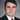# 03: Data Driven Programming

May 03, 2020

## LECTURES

### L14

2 types of expressions:

``````                 expr
_______________|___________________
|                                 |
atomic                           list
___|__________                     |
|            |            _______________________
self-eval    variables        procedures special-forms``````
``````    (define (eval exp)
(cond ((self-evaluating? exp) exp)
((symbol? exp) (look-up-global-value exp))
((special-form? exp) (do-special-form exp))
(else (apply (eval (car exp))
(map eval (cdr exp)) ))))``````

Above is the eval function for an interpreter in Scheme. Notice it attempts to determine the type of expression then has a function to handle them. If it is a list it recursively calls eval to break the list into primitives.

``````    (define (apply proc args)
(if (primitive? proc)
(do-magic proc args)
(eval (substitute (body proc) (formals proc) args))))``````

apply takes a procedure and a list of arguments. In the else of `eval` apply gets the first argument which will always the procedure call, and then it maps the arguments.

If the procedure is a primitive it gets handled at the machine language level.

If the procedure is created by a lambda, it has formal parameters and a body.

Scheme uses environmental model of evaluation, but we are building a substitution model interpreter right now. Substitution works for functional programming, but not all paradigms.

### L15

Why use a scheme interpreter to build a new one?

Helps understand model of evaluation
Experiment with modifications to scheme
Most of the original interpreter is written in scheme.
Conveys a big concept in cs which is universality.

universality is the concept of one machine that can run a large breadth of functions.

Applicative: argument values
Normal: argument expressions

Read: takes the input and turns it into box pointer diagrams.
Quote: takes cadr of the next expression.

### L16

concepts:
type tagging
data directed programming
message passing

type tagging: car is the symbol, cdr is the arguments.

data directed programming: write one operation that does everything.
Not functional programming if you call the same expression and get different results.

``````(get 'brian 'address)
; #f
; ok
; (781 soda)``````

This is not functional because same expression returns different results. You can still use these in a functional way by assigning at the beginning and never reassigning.

### L17

Horizontal slices through the table: messaging. The name of the interface knows all the function.

Horizontal slicing good for making prototypes.

4 kinds of numbers:
integer
rational
real
complex

regular people: 1 operand = unary, 2 operands = binary

## LAB

### lab 3

``````(define (count-change amount)
(cc amount '(50 25 10 5 1)))

(define (cc amount kinds-of-coins)
(cond ((= amount 0) 1)
((or (< amount 0) (equal? kinds-of-coins '())) 0)
(else (+ (cc amount
(cdr kinds-of-coins))
(cc (- amount
(first-denomination kinds-of-coins))
kinds-of-coins)))))

(define (first-denomination kinds-of-coins)
(car kinds-of-coins))``````

This modifies the procedure on page 40-41 for count-change.

This replaces the amount of coins with a sentence of coin amounts. I modified it to check for an empty array instead of 0, then when all possibilities of a denomination are checked I switch it to the remaining denominations using `cdr`. Then all I have to do to find the `first-denomination` is return `car` of the list.

### 2.4 Multiple Representations for Abstract Data

generic procedures: procedures that operate that may be represented in more than 1 way.

type tags allow for specificity. Telling the generic procedure how to operate for that specific type.

put: installs the item in the table
get: looks up the entry and returns item

### 2.5 Systems with Generic Operations

Coercion: Objects of one type may be viewed as being of another type.

Scheme checks to see if one type can be changed to another, if not it checks to see if the other can. If neither can change to the other it throws an error.

Types can have hierarchies. For example integers are a subtype of rational numbers. And rational numbers are a supertype of integers. Numbers have a simple hierarchy known as a tower. With integers on the bottom and complex numbers on the top.Software Engineer in Austin, Texas